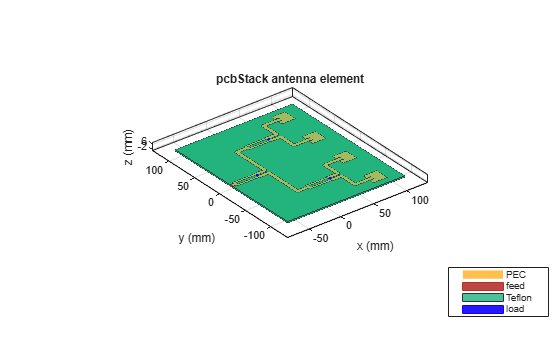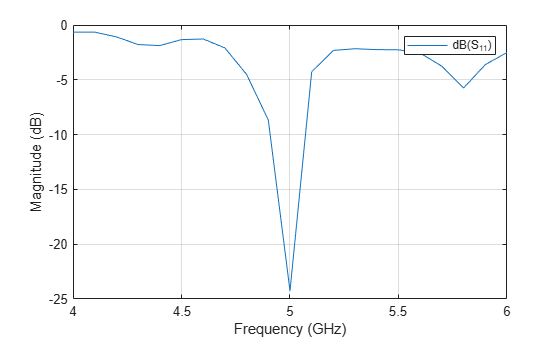# Corporate Feed Divider Network for a Linear Patch Antenna array

This example shows how to integrate a corporate power divider with a microstrip patch antenna array. The corporate power divider is available as a catalog element in RF PCB Toolbox. The patch antenna array is built using the `patchMicrostripInsetfed` catalog element and `pcbStack` from Antenna Toolbox.

### Create Variables

Create the common variables.

```f = 5e9; c = physconst('lightspeed');```

### Create Corporate Power Divider

Use the `powerDividerCorporate` object to design the corporate power divider at 5 GHz and adjust the spacing to wavelength. Use the `show` function to visualize it.

```pdc = powerDividerCorporate; pdc = design(pdc,f); pdc.SplitterElement.Substrate.LossTangent = 0; pdc.PortSpacing = c/f; pdc.PortLineLength = 10e-3; pdc.GroundPlaneWidth = pdc.PortSpacing*4; figure,show(pdc);```Use the `sparameters` function with `Behavioral` set to `true.P`lot s-parameters using the `rfplot` function. As the computation takes time, load the pre-computed mat file and plot the result using `rfplot`.

```% out = sparameters(pdc,linspace(4e9,6e9,31),'Behavioral',true); load pdc.mat figure; rfplot(sparPDC);```### Create Inset Fed Patch Antenna

Use the `patchMicrostripInsetfed `object to design a microstrip inset fed patch that resonates at 5 GHz.

```ant = patchMicrostripInsetfed; ant.Substrate = dielectric('Teflon'); ant = design(ant,5e9); ant.Height = 1.6e-3; ant.Length = 20.5e-3; ant.Width = 20.5e-3; ant.StripLineWidth = pdc.PortLineWidth; ant.NotchWidth = 7.1e-3; ant.NotchLength = 5.9e-3;```

Use the `sparameters` function to calculate the sparameters. As it takes more time to complete the simulation the pre-computed result is loaded.

`% out = sparameters(ant,linspace(4e9,6e9,31));`

Load the mat file and plot the sparameters using `rfplot` function.

```load patch.mat figure,rfplot(out);```### Create pcbStack for Antenna

Use the `pcbStack` object to convert the patch antenna into a pcb stack so that the shape of the top layer can be extracted from the `Layers` property. Convert into a linear array of 4 elements. The patch is extracted from the `Layers` property of the `pcbStack` and the shape is copied and converted into a linear array.

```pcbant = pcbStack(ant); TopLayer = pcbant.Layers{1}; TopLayer1 = copy(TopLayer); for i = 2:4 a = copy(TopLayer1); a = translate(a,[0,pdc.PortSpacing*(i-1),0]); TopLayer = TopLayer+a; end TopLayer = translate(TopLayer,[-ant.FeedLocation(1)+pdc.GroundPlaneLength/2,-pdc.PortSpacing*1.5,0]);```

### Create pcbComponent for Corporate Power Divider

Use the `pcbComponent` object and convert the corporate power divider into a PCB Component. Create a new `pcbStack `object to construct the cascade of power divider corporate and patch antennas. Assign all the properties of the pcbComponent to the pcbStack and then add the top layer of the corporate power divider with the patch antenna array and visualize it.

```pcbcomp = pcbComponent(pdc); pcbant1 = pcbStack; pcbant1.BoardShape = pcbcomp.BoardShape; pcbant1.BoardThickness =pcbcomp.BoardThickness; pcbant1.Layers = pcbcomp.Layers; pcbant1.FeedDiameter = pcbcomp.FeedDiameter; pcbant1.FeedViaModel = pcbcomp.FeedViaModel; pcbant1.FeedLocations = pcbcomp.FeedLocations; pcbant1.Load = pcbcomp.Load; a = pcbant1.Layers{1}; finalShape = a+TopLayer; pcbant1.Layers{1} = finalShape; feed = pcbant1.FeedLocations(1,:); pcbant1.FeedLocations = feed; gnd = pcbant1.Layers{3}; gnd.Length = gnd.Length+50e-3; gnd.Width = gnd.Width-20e-3; gnd.Center(1) = 50e-3/2; pcbant1.Layers{3} = gnd; pcbant1.BoardShape = gnd; figure,show(pcbant1);```Use the `mesh` function to manually mesh the structure and use the `sparameters` function to compute the s-parameters. Generating a mesh and computing the result takes a lot of time as the structure is large compared to the wavelength. Hence the lines are commented out.

```% figure,mesh(pcbant1,'MaxEdgeLength',6e-3); % spar = sparameters(pcbant1,linspace(4e9,6e9,21)); % figure,pattern(pcbant1,4.9e9)```

Load the precomputed result from `PDCpatch.mat` file and plot the results.

```load PDCpatch.mat figure; rfplot(spar)```Use the `patternCustom` function to plot the 2-D or 3-D radiation pattern of an antenna magnitude, `magE` over the specified `phi` and `theta` angle vectors.

```phi = az'; theta = (90-el); MagE = pat'; figure; patternCustom(MagE,theta,phi);```